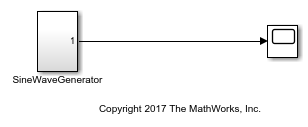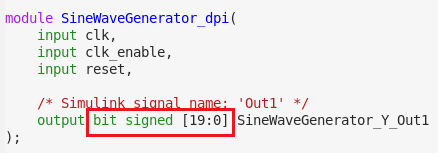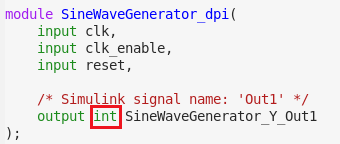# Generate Bit/Logic Vector Data Types

This example shows you how to generate bit or logic vector data types in the SystemVerilog interface of the DPI component. This capability is useful whenever having an exact width of the port is important to integrate the DPI component in your testbench.

### Requirements and Prerequisites

Products required for this example:

• MATLAB®

• Fixed-Point Designer™

• Mentor Graphics® ModelSim®/QuestaSim®

• One of the supported C compilers: Microsoft® Visual C++, or GNU GCC

### Overview

If the Simulink model has a port with fixed-point data type, by default, the generated SystemVerilog code maps the port to a compatible C type that can hold the necessary bits. For more information about these mappings, see "Supported Simulink Data Types" in the documentation.

This Simulink model generates a discrete sine wave using a signed fixed-point data type containing 20 bits. By default the generated SystemVerilog code uses 'int' data type to represent this port. This example shows how to generate SystemVerilog code that uses bit or logic vectors to represent the exact length of the fixed-point number.

### Open Example

Run the following code to open the design.

```open_system('svdpi_BitVector'); ```### Set Up Model for Code Generation

The model is preconfigured with one of the DPI-C system target files (systemverilog_dpi_grt.tlc). To generate SystemVerilog code with bit or logic vectors, open Configuration Parameters. Then, in Code Generation > System Verilog DPI, select "BitVector" from the "Fixed-point data type" menu.Alternatively, you can set this parameter by executing:

```set_param('svdpi_BitVector','DPIFixedPointDataType','BitVector'); ```

### Generate SystemVerilog DPI Component

1. In the "svdpi_BitVector" model, right click the SineWaveGenerator block, and select C/C++ Code -> Build This Subsystem.

2. Click Build in the dialog box that appears.

3. The build generates C code for the SineWaveGenerator subsystem, a System Verilog DPI wrapper "SineWaveGenerator_build/SineWaveGenerator_dpi.sv", and a package file "SineWaveGenerator_build/SineWaveGenerator_dpi_pkg.sv".

Alternatively you can generate the component by executing:

```rtwbuild('svdpi_BitVector/SineWaveGenerator'); ```

### Inspect the SystemVerilog Code

Open the DPI component "SineWaveGenerator_dpi.sv" and note the data type at the interface:If you select "Logic Vector" then the SystemVerilog DPI-C component uses the SystemVerilog "logic" type for the interface.

If you choose the default "Compatible C Types", then the generated SystemVerilog data type is "int" as shown:### Considerations for Port Types

• "Compatible C Types" supports ports up to 64 bits wide. If your design contains ports with word length greater than 64, then you must select "Bit Vector" or "Logic Vector".

• If your design contains a vector of fixed-point numbers and you select "Bit Vector" or "Logic Vector" then the resulting data type is a mixed (unpacked and packed) SystemVerilog array. For example a vector of size 3 containing fixed-point numbers of word length 2 would map to bit [1:0] PortName .

## SupportGet trial now